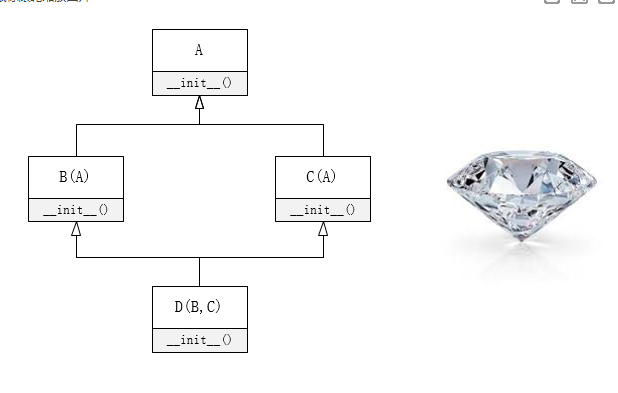# [扩展阅读] 多重继承的陷阱：钻石继承（菱形继承）问题

## 多重继承的陷阱：钻石继承（菱形继承）问题：

class A():
def __init__(self):
print("进入A…")
print("离开A…")

class B(A):
def __init__(self):
print("进入B…")
A.__init__(self)
print("离开B…")

class C(A):
def __init__(self):
print("进入C…")
A.__init__(self)
print("离开C…")

class D(B, C):
def __init__(self):
print("进入D…")
B.__init__(self)
C.__init__(self)
print("离开D…")

----------------------------------------------------------------------------------------
d = D()
========================= RESTART: D:/python/fish.py =========================
>>> d = D()>>> D.__mro__
(<class '__main__.D'>, <class '__main__.B'>, <class '__main__.C'>, <class '__main__.A'>, <class 'object'>)

class A():
def __init__(self):
print("进入A…")
print("离开A…")

class B(A):
def __init__(self):
print("进入B…")
super().__init__()
print("离开B…")

class C(A):
def __init__(self):
print("进入C…")
super().__init__()
print("离开C…")

class D(B, C):
def __init__(self):
print("进入D…")
super().__init__()
print("离开D…")

>>> d = D()